Transfer Function Next  |  Prev  |  Up  |  Top  |  Index  |  JOS Index  |  JOS Pubs  |  JOS Home  |  Search

## Transfer Function

As we cover in Chapter 6, the transfer function of a digital filter is defined as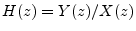where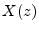is the z transform of the input signal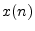, and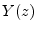is the z transform of the output signal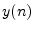. We may find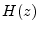from Eq. (3.1) by taking the z transform of both sides and solving for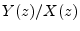: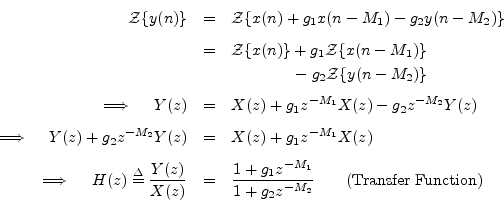Some principles of this analysis are as follows:

1. The z transform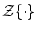is a linear operator which means, by definition,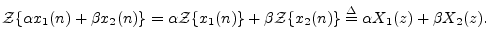2.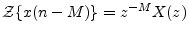. That is, the z transform of a signaldelayed by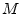samples,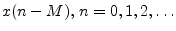, is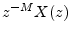. This is the shift theorem for z transforms, which can be immediately derived from the definition of the z transform, as shown in §6.3.2.
Note that these two properties of the z transform are all we really need to find the transfer function of any linear, time-invariant digital filter from its difference equation (its implementation formula in the time domain).

In matlab, difference-equation coefficients are specified as transfer-function coefficients (vectors B and A in Fig.3.5). This is why a minus sign is needed in Eq. (3.3).

Ok, so finding the transfer function is not too much work. Now, what can we do with it? There are two main avenues of analysis from here: (1) finding the frequency response by setting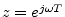, and (2) factoring the transfer function to find the poles and zeros of the filter. One also uses the transfer function to generate different implementation forms such as cascade or parallel combinations of smaller filters to achieve the same overall filter. The following sections will illustrate these uses of the transfer function on the example filter of this chapter.

Next  |  Prev  |  Up  |  Top  |  Index  |  JOS Index  |  JOS Pubs  |  JOS Home  |  Search

[How to cite this work] [Order a printed hardcopy]